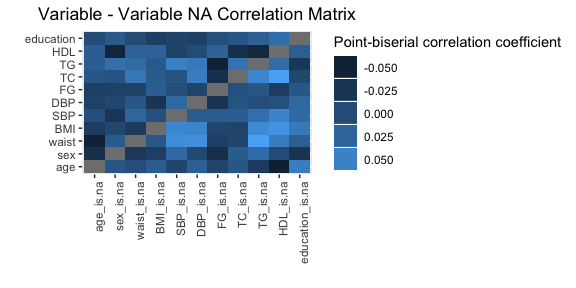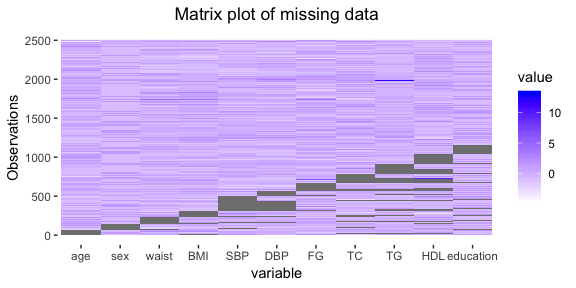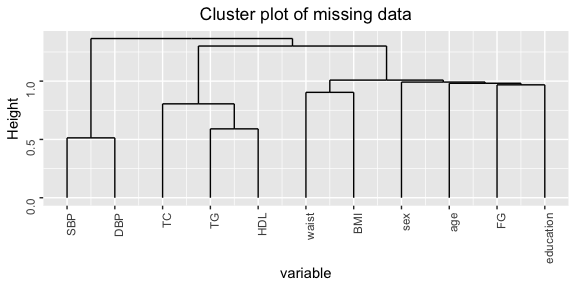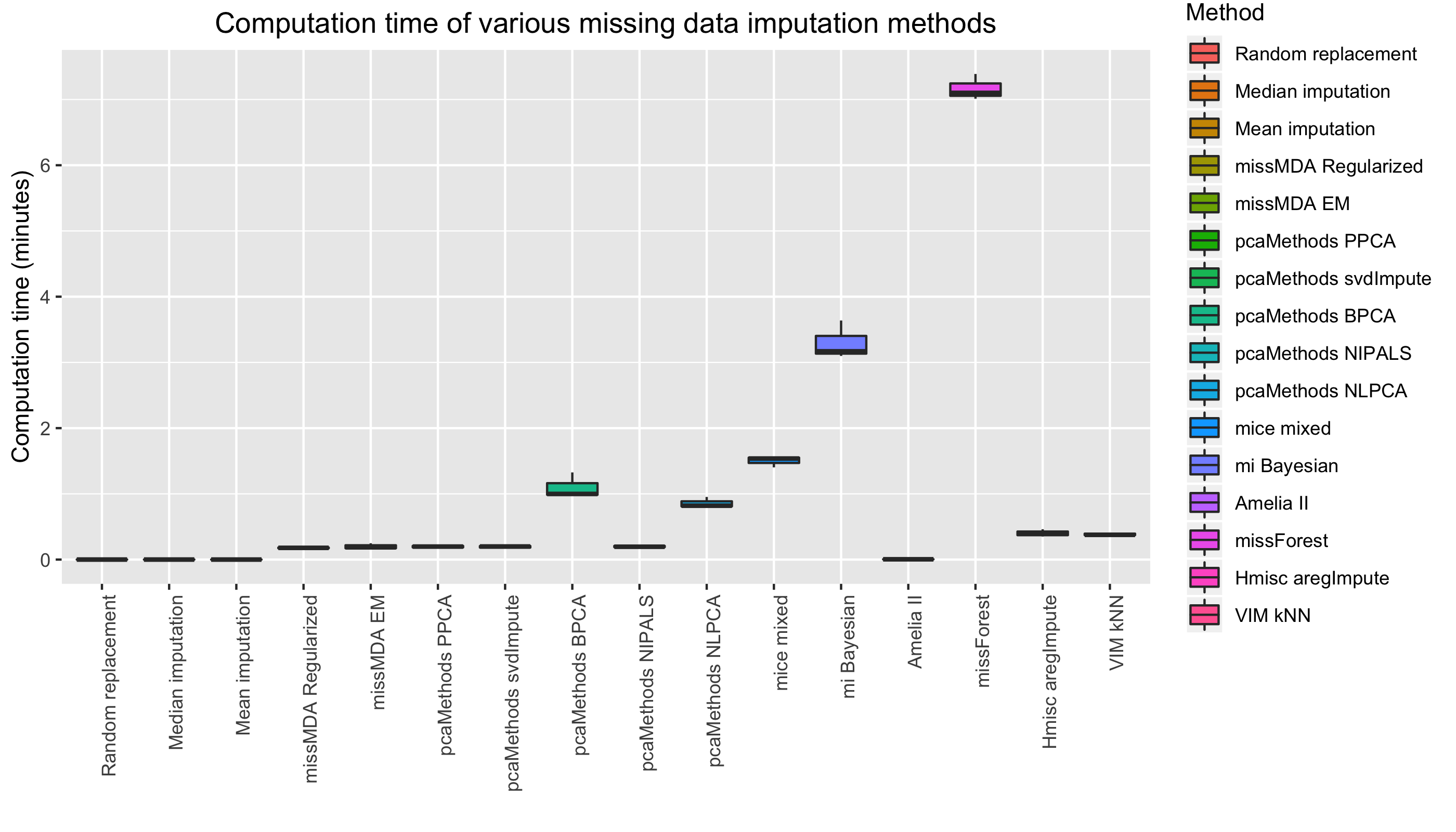# A complete tutorial to missCompare

#### 2019-01-31

If you work with data, you have almost certainly encountered issues regarding missing data. The most commonly used statistical methods often only work with complete sets of observations and it can be a huge pain when you realize that you can only utilize a fraction of your data because of missing data points here and there. Indeed, just 5% random missing data in a huge dataset can dramatically reduce the number of complete observations to as low as 25% of the original number of observations! Scary! Thankfully, there are numerous algorithms that can help you tackle this issue, but often, the most commonly used methods, such as mean and median imputation, offer suboptimal solutions and sophisticated algorithms often need a lot of consideration and background knowledge. The authors wrote the missCompare package to help you through your missing data problem. The initial hypothesis of the authors was that datasets with different parameters (size, distributions, correlations, etc.) will benefit differently from various missing data imputation algorithms.

This tutorial will navigate you through the missCompare package and its functions. So… let’s jump right into it!

``````install.packages("missCompare")
library(missCompare)``````

## Description of data: clindata_miss

To walk you through the missCompare missing data imputation pipeline, the authors created the `missCompare::clindata_miss` dataframe. As the name suggest, clindata_miss contains missing data. You can load this dataframe into your workspace by:

``data("clindata_miss")``

The data is created by the authors with the purpose of generating a dataset that resembles real-life data with variables generally available in a simple, realistic clinical epidemiology dataset. This R dataframe have realistic variable means, distributions, correlations and missing data patterns. You can find further information on the dataframe using `?clindata_miss`.

## Before you start…

…Some words on alternative imputation methods. Before you get yourself acquainted with missCompare and its functions, it is always a good idea to carefully observe, and do some thinking about your data, your variables/features and your missing data. Before trying fancy methods, there are some cases when deductive imputation can be applied. This is a form of imputation based on logical reasoning rather than statistical methods. Let’s consider three separate cases when this can be applied:

1. Constant variables: In this scenario, in a prospective dataset, sex information is available at a participant’s first visit in 1998 and third visit in 2005, but missing in 2000. With logical deduction, it can be concluded that biological sex is constant, therefore the missing data point is also Female

ID Date Sex BMI
ID13 01-01-1998 Female 25.8
ID13 01-01-2000 NA 27.7
ID13 01-01-2005 Female 27.0
2. Prospective changes: In this scenario, in a prospective dataset, age information is available at a participant’s first visit in 1998, but missing in 2000 and 2005. With logical deduction, it can be concluded that missing age information is 25 and 30 for the missing two years

ID Date Age BMI
ID13 01-01-1998 23 25.8
ID13 01-01-2000 NA 27.7
ID13 01-01-2005 NA 27.0
3. Cumulative variables: In this scenario, in a prospective dataset, there is information available on how many times the participant has been pregnant. This information is available at a participant’s last visit in 2005, but missing in 1998 and 2000. With logical deduction, it can be concluded that if the participant has never been pregnant in 2005, than she has never been pregnant earlier either, therefore the missing numbers are 0 and 0

ID Date Number of pregnancies BMI
ID13 01-01-1998 NA 25.8
ID13 01-01-2000 NA 27.7
ID13 01-01-2005 0 27.0
4. Dependent variables / known formula: In some cases, there could be a known relationship between variables. Such case is when the variables height, weight and BMI are present in the data, and some values are randomly missing. As BMI is calculated as weight (kg) /height2 (m2), knowing the formula it can be deducted that the values below are 70 kg and 31.2 kg/m2. A similar scenario in clinical datasets would be missing laboratory lipid values, where LDL cholesterol values are calculated by the Friedewald formula from triglycerides, total cholesterol and HDL cholesterol values. Another classic scenario is linearly dependent variables, where the sum of two variables produce a third variable.

ID Weight_kg Height_meters BMI
ID13 NA 1.65 25.8
ID15 74 1.78 23.4
ID16 89 1.69 NA

All of the above scenarios need prior knowledge of the data, which is “easy” for humans, but is very hard to compute into any single missing data imputation algorithm. Therefore, it is always suggested that the variables and their relationships are thoroughly understood before jumping into hardcore data imputation using a fancy algorithm.

Also, it is highly recommended to check data ranges. By running a simple `summary()` on your data you can check minimum and maximum values for each numeric variables and browse through all categories for factors. Oftentimes it happens that a variable - a blood biomarker, for instance - has a minus value as its minimum. While this can be OK in some cases (e.g. the variable is already normalized), but sometimes this can be a data input error or missing data points can be coded as minus values. In these cases, it is important that the minus values are set to NA.

Another important aspect that should be considered are outliers. This tutorial is not meant to delve too deep into this, but it is suggested that outliers are visualized for each variables and are excluded if they are too extreme or represent non-sense values. E.g. an age value of 109 is definitely an outlier, but physiologically possible, but 309 is probably due to a data input error, therefore it should be set to NA.

## The missCompare pipeline

The missCompare pipeline is comprised of the following steps:

1. Cleaning your data using `missCompare::clean()`
2. Extracting information on dimensions, missingness, correlations and variables, plotting missing data using `missCompare::get_data()`
3. Imputation - simulated data:
• simulating full data with no missingness using metadata from the previous step (resembling your original data) using `missCompare::simulated()`
• spiking in missing data in distinct missing data patterns using `missCompare::all_patterns()`. These patterns are:
• missing completely at random (MCAR) - `missCompare::MCAR()` - missing data occurrence random
• missing at random (MAR) - `missCompare::MAR()` - missing data occurrence correlates with other variables’ values
• missing not at random (MNAR) - `missCompare::MNAR()` - missing data occurrence correlates with variables’ own values
• missing in assumed pattern (MAP) - `missCompare::MAP()` - a combination of the previous three, where the user can define a pattern per variable
• imputing missing data, obtaining measures of imputation quality (root mean squared error - RMSE; mean absolute error - MAE; Kolmogorov–Smirnov test statistic - KS; computation time) per method and plotting results using `missCompare::impute_simulated()`
1. Imputing your data - After the previous step, you will have a general idea on what are the best performing algorithms for your data structure (size, degree of correlation between variables). In this step you can impute your original data with your chosen algorithm(s) using `missCompare::impute_data()`
2. Post imputation diagnostics will give an informative assessment on how the imputation changed your data structure (e.g. variable means, distributions, correlations). The function here is `missCompare::post_imp_diag()`.

Now let’s look into these, step by step!

## Cleaning and getting to know the data

The first step before starting anything imputation related is getting to know your data and cleaning it if necessary. This is not an “automated” process, nor should it be. You always need to explore your data before missing data imputation - it is entirely up to you what to keep and what to drop from your dataset before the imputation process. After the cleaning steps, missCompare will essentially reconstruct your dataset with no missing data, and in order to achieve this, your dataframe cannot have variables of type character or factor (in a later stage you will be able to use your original factor variables, but to extract metadata at this step, a conversion is necessary).

Let’s take clindata_miss and run `missCompare::clean()`. In one step, you can achieve multiple cleaning steps:

``````cleaned <- missCompare::clean(clindata_miss,
var_removal_threshold = 0.5,
ind_removal_threshold = 0.8,
missingness_coding = -9)
#> Variable(s) sex, education converted to numeric.
#> Variable(s) PPG removed due to exceeding the pre-defined removal threshold (>50%) for missingness.
#> 1 individual(s) removed due to exceeding the pre-defined removal threshold (>80%) for missingness.``````
• You can convert all factor variables (sex and education, in this case) to numeric. The function will alert you with a message: “Variable(s) sex, education converted to numeric.”
• You can remove variables exceeding a set threshold (default is 50%, but this is up to you, you can set it lower or higher). In other words, you can discard those variables where lots of data are missing. Such variables can only be imputed with low accuracy, so the authors suggest that you remove variables with high missingness fraction, but there is no recipe for this, e.g. you will find no guidelines on whether 50% is too high or too low, the threshold will be YOUR decision. In case there is a variable removed here, the function will alert you with a message: Variable(s) PPG removed due to exceeding the pre-defined removal threshold (>50%) for missingness.
• Similarly, you can remove individuals with a high percentage of missing values (default is 100%, but this is up to you, you can set it lower). In other words, you can discard those observations that have only missing data points (these will be totally uninformative for imputation), or those with lots of missing data points. Again, there are no guidelines here, the threshold is ultimately YOUR decision. In this case, we set this threshold to 0.8, and the function alerted us with a message: 1 individual(s) removed due to exceeding the pre-defined removal threshold (>80%) for missingness.
• In some datasets, missing values can be coded in a weird way. In clindata_miss, we emulated a real-life scenario where lipid values were set to -9 when measured below the sensitivity of a hypothetical machine. The cleaning function will let you convert pre-defined values to NAs.

The cleaning function outputs a dataframe called `cleaned`. In the next step, where you extract important metadata from your dataframe, you will continue working with this object.

You will get to know important details of your cleaned dataframe using the following line:

``````metadata <- missCompare::get_data(cleaned,
matrixplot_sort = T,
plot_transform = T)``````

If there are non-numeric variables in the data, a warning will appear: Warning! Variable(s) sex, education is/are not numeric. Convert this/these variables to numeric using missCompare::clean() and repeat the missCompare::get_data() function until no warnings are shown.

As we cleaned clindata_miss in the previous step, the function should run without any warnings and errors. The output object, `metadata` is a list of the following elements.

• `metadata\$Complete_cases` - the number of complete observations with no missing data. In this case, there are 1349 observations from the original 2500 with no missing data. Note, that with only ~7% missing data, the number of complete observations is dramatically decreased to around half of the original data. As many methods (e.g. regression, principal component analysis, etc.) require complete sets of observations, this decrease in complete observations presents a very strong case for missing data imputation.
• `metadata\$Rows` and `metadata\$Columns` give you the dimensions of the dataframe, 2499 rows and 11 columns, in our case.
• `metadata\$Corr_matrix` - gives you the correlation matrix of all variables in the dataframe. The function assesses pairwise Pearson correlation coefficients using complete sets of pairs between any two variables. For example there are 2312 complete BMI-waist pairs (`sum(!is.na(cleaned\$BMI) & !is.na(cleaned\$waist))`) - in this case correlation will be calculated based on these 2312 value pairs, even though the number of complete observations (no NAs for any variables) is 1349. This way all of the data will be utilized to obtain the most accurate correlation coefficients.
• `metadata\$Fraction_missingness` and `metadata\$Fraction_missingness_per_variable` return the the total fraction of missingness in the dataframe and the fraction of missingness per variable, respectively. In our case, the total fraction of missingness is 6.9% after removing one variable, PPG with >50% missing. Inspecting the fraction of missingness per variable it is also apparent that the true missing data fraction of TG and HDL-C after converting -9s to NAs are 10.6% and 13.7%, respectively.
• `metadata\$Total_NA` and `metadata\$NA_per_variable` return the total number of missing values in the dataframe and per variable.
• `metadata\$MD_Pattern` - The missing data pattern is calculated using mice::md.pattern (`?mice::md.pattern`).
• `metadata\$NA_Correlations` - Pairwise correlation point-biserial correlation coefficients between variable pairs. Namely, for each calculation, variables are converted to TRUE/FALSE based on missing data status (missing or not missing) and these boolean vectors and correlated to the native variables. The resulting correlation matrix has rows with variable names + _is.na - the rows represent the converted variables, while the columns are the original variables.
• `metadata\$NA_Correlation_plot` - A correlation plot based on the previous object, `metadata\$NA_Correlations`.• `metadata\$min_PDM_thresholds` - This object is a small dataframe that informs about the various min_PDM (minimum number of observations per distinct missing data pattern) values. min_PDM will need to be defined in further functions, so it is informative to get a sense on what is the proportion of missing data patterns that should be used in the simulation framework. In the table below we see that if this number is too high (only those missing data patterns are considered that describe 100 or more observations) than more than 80% of observations with other missing data patterns will be ignored in the simulation framework. On the other hand, if this number is set too low, e.g. below 5, than this may result in retaining almost all missing data patterns that are applicable to only a few observations resulting in a very complicated pattern that the algorithm might fail to apply to your dataset. Therefore, this number will need to be set carefully later. This table shows approximate percentages of observations with missing data retained with setting min_PDM in later steps.
``````#>   min_PDM_threshold perc_obs_retained
#> 1                 5                82
#> 2                10                78
#> 3                20                75
#> 4                50                53
#> 5               100                19
#> 6               200                 0
#> 7               500                 0
#> 8              1000                 0``````
• `metadata\$Vars_above_half` - In case there are variables exceeding 50% missingness, this object will list these variables and the function will output a warning: Warning! Missingness exceeds 50% for variable(s) PPG. Although the pipeline will function with variables with high missingness, consider excluding these variables using missCompare::clean() and repeating function until no warnings are shown.
• `metadata\$Matrix_plot` - Matrix plot (ggplot2 object) visualizing missing data distribution. Missing data are represented by gray color, while data is colored by value. There are options to sort the dataframe by missingness or leave the dataframe unsorted. This plot (hopefully) gives you a visual description of how data is missing together and what fraction of the data is missing.• `metadata\$Cluster_plot` - Dendrogram (ggplot2 object) visualizing the hierarchical clustering of missing data. On the plot, the clades (bifurcations) closer to Height 0 (the bottom of the plot) represent closer relationships in terms of missing data - i.e. those variables in one group are more likely to be missing together compared to the rest. In our case, blood pressures SBP and DBP are missing together often and so as the three lipid traits, TC, TG and HDL-C. With respect to the other variables, waist and BMI have a closer relationship compared to the rest.## Simulating a complete dataset and assessing algorithms

Having learned the characteristics of your data, the next step is to assemble a framework in which you can test the performance of various missing data algorithms on simulated data. This can be achieved in one step using `missCompare::impute_simulated()`, but let’s look under the hood and see how this function is operating in the background. You can access these functions in case you would like to play with parameters.

First, `missCompare::simulate()` will create a dataset that resembles your original dataframe, but with no missing data. The dimensions of the simulated dataframe and all correlations between variables will match the original data - essentially, the only major difference between the simulated dataset and your original data is that here, all variables are normally distributed with a custom set mean and standard deviation. Let’s observe:

``````simulated <- missCompare::simulate(rownum = metadata\$Rows,
meanval = 0,
sdval = 1)``````

Note how the first three arguments are elements of the output list (`metadata`) from the previous function `missCompare::get_data()`.

The output `simulated` is a list, where the first item is the simulated matrix and the next two objects are samples from the correlation matrix of the original and the simulated data.

With this done, missing data can be spiked in in the simulated data using various patterns (MCAR, MAR, MNAR and MAP). This will be done with respect to the missing data fraction for each variable in the original dataframe.

MCAR missingness is simple, NAs are spiked in randomly. Please note that in all functions below, min_PDM is set to 10. As it was shown before when looking at outputs from `missCompare::get_data()`, this will result in the retention of the most important missing data patterns in which approximately 78% of all observations will be accounted for.

``````missCompare::MCAR(simulated\$Simulated_matrix,
min_PDM = 10)``````

MAR and MNAR missingness follow similar logic. With MNAR, NAs are spiked in based on the variable’s own values, while with MAR, NAs are spiked in based on all other variables’ values.

``````missCompare::MAR(simulated\$Simulated_matrix,
min_PDM = 10)

missCompare::MNAR(simulated\$Simulated_matrix,
min_PDM = 10)``````

MAP missingness is a possible combination of any of the previous three missing data pattern. You can define what pattern you assume per variable. In our case, let’s say that all variables are missing randomly, except for education (the last variable), which is missing not at random. This can be defined as:

``````missCompare::MAP(simulated\$Simulated_matrix,
min_PDM = 10,
assumed_pattern = c(rep("MCAR", 10), "MNAR"))``````

All patterns can be run with a single line using `missCompare::all_patterns()`.

Again, `missCompare::impute_simulated()` does all these in one step, and more:
1. it simulates the dataset with no missing values;
2. spikes in NAs in MCAR, MAR, MNAR and - if you define a custom pattern - MAP patterns;
3. imputes missing data using a curated list of 16 algorithms;
4. compares computation time, evaluates imputation accuracy by calculating RMSE, MAE and KS values between the imputed and the original data points.

This all can be achieved by a single command! This means you DON’T have to run `missCompare::simulate()`, `missCompare::MCAR()`, `missCompare::MAR()`, `missCompare::MNAR()`, `missCompare::MAP()` or `missCompare::all_patterns()`. The command goes as (note that we defined `assumed_pattern = NA` - the default - so MAP pattern will be skipped):

``````missCompare::impute_simulated(rownum = metadata\$Rows,
min_PDM = 10,
n.iter = 50,
assumed_pattern = NA)``````

The `n.iter` argument controls how many times the process will repeat itself (spiking in missing data, imputation and assessment of imputation accuracy, that is).

When this is completed (depending on the size of you data, it can take some time!), you should get messages stating what are the best performing algorithms for each missingness patterns (evaluated by various metrics) and a graph that gives you a visual aid on which algorithms perform in the most desired fashion. The less the error, and the more the imputed values’ distributions match the original values’ distributions, the better - i.e. lower RMSE, MAE and KS D statistic values are better! Computation time of course can also play a role in your decision on what to use eventually!

Let’s look at some plots:

## Computation time comparison